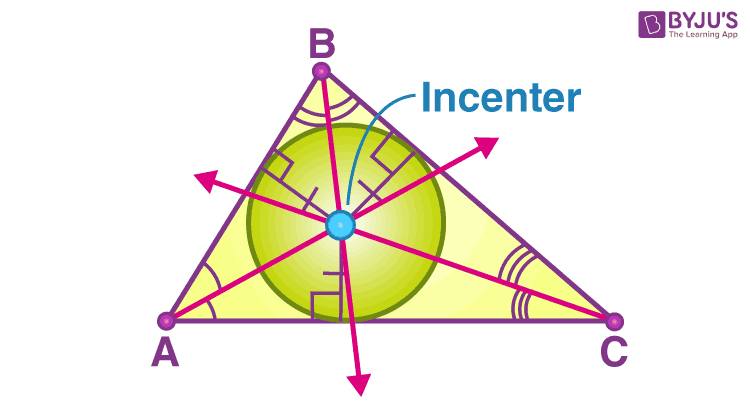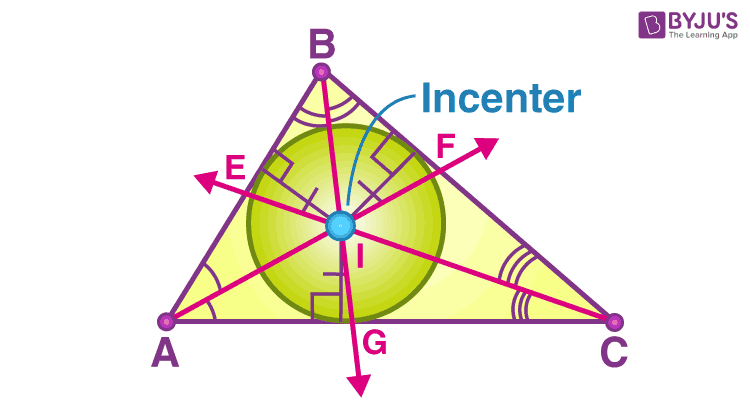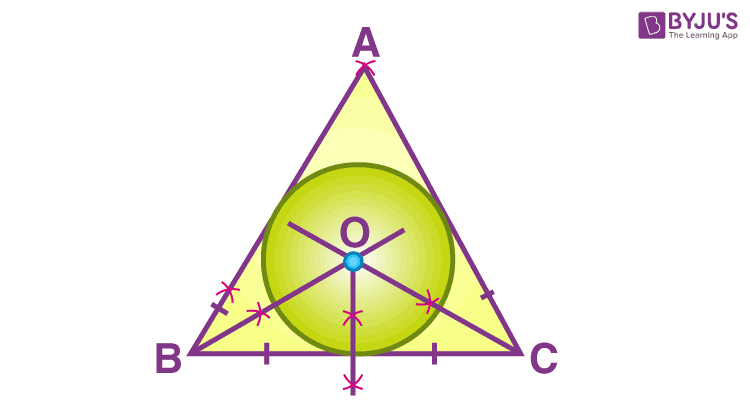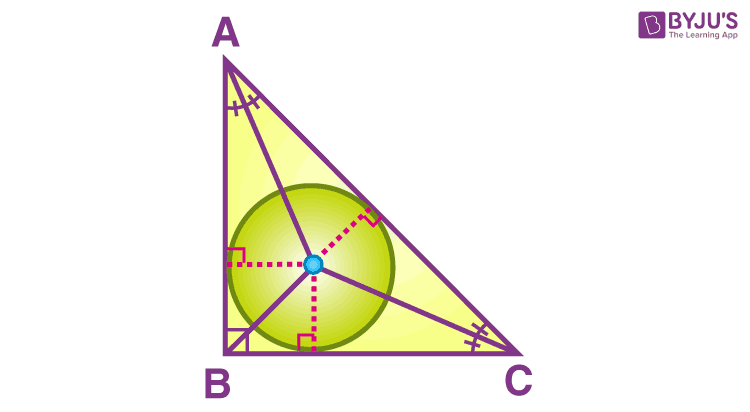# Incenter of a Triangle

In geometry, a triangle is a type of two-dimensional polygon, which has three sides. When the two sides are joined end to end, it is called the vertex of the triangle. An angle is formed between two sides. This is one of the important elements of geometry. Triangles possess different properties, and each of these properties can be studied at different levels of education. In this article, you will understand what is the incenter of a triangle, formula, properties and examples.

## Incenter of a triangle Meaning

The incenter of a triangle is the intersection point of all the three interior angle bisectors of the triangle. In other words, it can be defined as the point where the internal angle bisectors of the triangle cross. This point will be equidistant from the sides of a triangle, as the central axis’s junction point is the centre point of the triangle’s inscribed circle.

The incenter of a triangle is the center of its inscribed circle which is the largest circle that will fit inside the triangle. This circle is also called an incircle of a triangle. This can be observed from the below figure.## Incenter of a Triangle Formula

Suppose (x1, y1), (x2, y2) and (x3, y3) are the coordinates of vertices of a triangle ABC and a, b and c are the lengths of its sides, then the triangle’s incenter can be calculated using the formula:

$$\LARGE (\frac{ax_1+bx_2+cx_3}{a + b + c},\ \frac{ay_1+by_2+cy_3}{a + b + c})$$

The above formula helps in solving the problems like How to find the incenter of a triangle with 3 coordinates.

To solve such problems, we can just substitute the coordinates in the formula after finding the lengths of sides of a triangle using the distance formula in coordinate geometry.

### Incenter of a Triangle Angle Formula

Let E, F and G be the points where the angle bisectors of C, A and B cross the sides AB, AC and BC, respectively.Using the angle sum property of a triangle, we can calculate the incenter of a triangle angle.

In the above figure,

∠AIB = 180° – (∠A + ∠B)/2

Where I is the incenter of the given triangle.

### Incenter of a Triangle Properties

Below are the few important properties of triangles’ incenter.

1. If I is the incenter of the triangle ABC (as shown in the above figure), then line segments AE and AG, CG and CF, BF and BE are equal in length, i.e. AE = AG, CG = CF and BF = BE.
2. If I is the incenter of the triangle ABC, then ∠BAI = ∠CAI, ∠BCI = ∠ACI and ∠ABI = ∠CBI (using angle bisector theorem).
3. The sides of the triangle are tangents to the circle, and thus, EI = FI = GI = r known as the inradii of the circle or radius of incircle.
4. If s is the semiperimeter of the triangle and r is the inradius of the triangle, then the area of the triangle is equal to the product of s and r, i.e. A = sr.
5. The triangle’s incenter always lies inside the triangle.

### How to Find Incenter of a Triangle

There are two different situations in which we have to find the triangles’ incenter. In construction, we can find the incenter, by drawing the angle bisectors of the triangle. However, in coordinate geometry, we can use the formula to get the incenter.

Let’s understand this with the help of the below examples.

Example 1: Find the coordinates of the incenter of a triangle whose vertices are given as A(20, 15), B(0, 0) and C(-36, 15).

Solution:

Let the given points be:

A(20, 15) = (x1, y1)

B(0, 0) = (x2, y2)

C(-36, 15) = (x3, y3)

Using distance formula we can find the length of sides AB, BC and CA as:

AB = c = √[(20 – 0)2 +(15 – 0)2] = 25

BC = a = √[(0 + 36)2 + (0 – 15)2] = 39

CA = b = √[(20 + 36)2 + (15 – 15)2] = 56

Substituting these values in incenter formula,

$$(\frac{ax_1+bx_2+cx_3}{a + b + c},\ \frac{ay_1+by_2+cy_3}{a + b + c})$$

= [(780 + 0 -900)/(39 + 56 + 25), (585 + 0 + 375)/(39 + 56 + 25)]

= (-120/120, 960/120)

= (-1, 8)

Example 2: Using ruler and compasses only, draw an equilateral triangle of side 5 cm and its incircle.

Solution:### Incenter of a Right Triangle

The incenter of a right angled triangle is given in the below diagram.